#### Nondeterministic case

Suppose that the nondeterministic model of nature is used. A dynamic programming recurrence, (10.39), will be derived. This directly yields an iterative approach that computes a plan that minimizes the worst-case cost. The following presentation shadows that of Section 2.3.1; therefore, it may be helpful to refer back to this periodically.

An optimal plan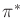will be found by computing optimal cost-to-go functions. For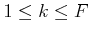, let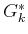denote the worst-case cost that could accumulate from stageto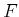under the execution of the optimal plan (compare to (2.5))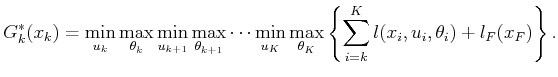(10.33)

Inside of the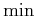's and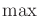's of (10.33) are the last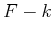terms of the cost functional, (10.8). For simplicity, the ranges of eachand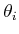in the's and's of (10.33) have not been indicated. The optimal cost-to-go for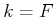is(10.34)

which is the same as (2.6) for the predictable case.

Now consider making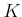passes over, each time computingfrom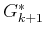, asranges fromdown to. In the first iteration,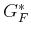is copied from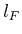. In the second iteration,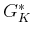is computed for each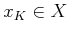as (compare to (2.7))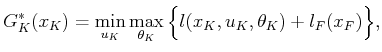(10.35)

in which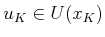and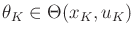. Since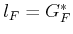and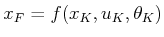, substitutions are made into (10.35) to obtain (compare to (2.8))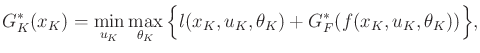(10.36)

which computes the costs of all optimal one-step plans from stageto stage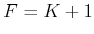.

More generally,can be computed onceis given. Carefully study (10.33), and note that it can be written as (compare to (2.9))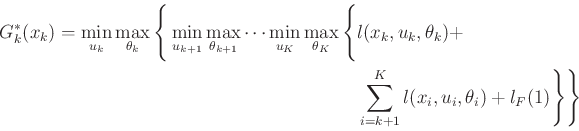(10.37)

by pulling the first cost term out of the sum and by separating the minimization over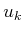from the rest, which range from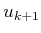to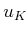. The secondanddo not affect the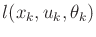term; thus,can be pulled outside to obtain (compare to (2.10))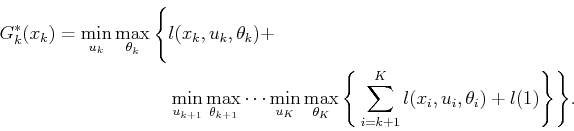(10.38)

The inner's and's represent, which yields the recurrence (compare to (2.11))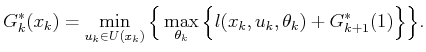(10.39)

Steven M LaValle 2020-08-14